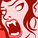# QlikView App Dev

Discussion Board for collaboration related to QlikView App Development.

Announcements
We value your feedback – take our 5-minute QlikView modernization survey
cancel
Showing results for
Did you mean:Partner - Contributor II

## Conditional Where clause in Load Script

Hi,

I'm trying to work out how to do a conditional where clause in a Load Script, does anyone have any ideas?

Previously in my Load Script, I have defined a variable, let's call it vType.

I know that I can do the load in the following way:

IF vType = 1 THEN

TABLE:

,FIELD_2

,FIELD_3;

SQL

SELECT FIELD_1

,FIELD_2

,FIELD_3

FROM TABLE;

ELSE

IF vType = 2 THEN

TABLE:

,FIELD_2

,FIELD_3

WHERE NOT EXISTS(FIELD_1);

SQL

SELECT FIELD_1

,FIELD_2

,FIELD_3

FROM TABLE;

ENDIF

ENDIF

But is there anyway that I can do this in one loadscript? The only difference that vType gives is that:

* vType = 1 => No where exists clause

* vType = 2 => Specific where exists clause

I was wondering if there was some way that I can set a variable to do this but I can't seem to do it successfully. The reason for wanting to do this is that the loadscript up until that point will always be exactly the same and in the case of long load scripts, this saves the need and potential human error of having two identical load scripts needed with the only difference being the existence of a where exists clause.

I guess effectively what I'm thinking is that there must be some way to do it in some way along these lines (although this doesn't work):

IF vType = 1 THEN

SET vWhereClause = '';

ELSE

IF vType = 2 THEN

SET vWhereClause = 'WHERE EXISTS(FIELD_1)';

ENDIF

ENDIF

TABLE:

,FIELD_2

,FIELD_3

'\$(vWhereClause);

SQL

SELECT FIELD_1

,FIELD_2

,FIELD_3

FROM TABLE;

1 Solution

Accepted SolutionsPartner - Specialist III

Hi Jamie,

I understand you have it correct in logic with your code

```IF vType = 1 THEN
SET vWhereClause =;
ELSE
IF vType = 2 THEN
SET vWhereClause = WHERE EXISTS(FIELD_1);
ENDIF
ENDIF

TABLE:
,FIELD_2
,FIELD_3
\$(vWhereClause);
SQL
SELECT FIELD_1
,FIELD_2
,FIELD_3
FROM TABLE;
```

But i removed the apostrophes on the set statements and on the the \$() expansion.

Should work this way as I see it, since set already treats it as a string, and when expanding, your expanding it to 'WHERE EXISTS(FIELD_1)'

The following code loads up specific rows, based on the first table:

```EXISTS:
[
FIELD_TEST
1
2
3
];

DATA:
[
FIELD_1,FIELD_2,FIELD_3
1,a,100
2,a,100
3,b,100
4,c,100
5,a,1000
];

let vType = 1;

IF vType = 1 THEN
SET vWhereClause =;
ELSE
IF vType = 2 THEN
SET vWhereClause = WHERE EXISTS(FIELD_TEST,FIELD_1);
ENDIF
ENDIF

NoConcatenate
TABLE:
FIELD_2,
FIELD_3
\$(vWhereClause);
RESIDENT DATA;

drop table DATA;
```

If you change the vType equal to 2 (two), you'll only load up the rows with FIELD_1 equal to 1,2 or 3.

3 RepliesPartner - Specialist III

Hi Jamie,

I understand you have it correct in logic with your code

```IF vType = 1 THEN
SET vWhereClause =;
ELSE
IF vType = 2 THEN
SET vWhereClause = WHERE EXISTS(FIELD_1);
ENDIF
ENDIF

TABLE:
,FIELD_2
,FIELD_3
\$(vWhereClause);
SQL
SELECT FIELD_1
,FIELD_2
,FIELD_3
FROM TABLE;
```

But i removed the apostrophes on the set statements and on the the \$() expansion.

Should work this way as I see it, since set already treats it as a string, and when expanding, your expanding it to 'WHERE EXISTS(FIELD_1)'

The following code loads up specific rows, based on the first table:

```EXISTS:
[
FIELD_TEST
1
2
3
];

DATA:
[
FIELD_1,FIELD_2,FIELD_3
1,a,100
2,a,100
3,b,100
4,c,100
5,a,1000
];

let vType = 1;

IF vType = 1 THEN
SET vWhereClause =;
ELSE
IF vType = 2 THEN
SET vWhereClause = WHERE EXISTS(FIELD_TEST,FIELD_1);
ENDIF
ENDIF

NoConcatenate
TABLE:
FIELD_2,
FIELD_3
\$(vWhereClause);
RESIDENT DATA;

drop table DATA;
```

If you change the vType equal to 2 (two), you'll only load up the rows with FIELD_1 equal to 1,2 or 3.Creator III

Hi,

use following expression :

SET vWhereClause =  pick(wildmatch(vType,1,2),'','WHERE EXISTS(FIELD_1)')

Use the \$(vWhereClause) as where condition shown abovePartner - Contributor II
Author

Thanks to both of you for the help, it seemed to work as expected!

I have a question of curiosity Jayant, I made use of this expression but why would you use wildmatch over match in this instance?